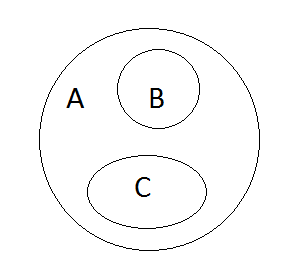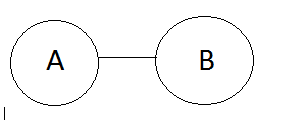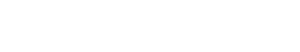Syllogism is one of the most important and interesting topics in Reasoning and Solving Syllogism is always fun if you understand the shortcuts. If you understand the below concepts I assure you that you can solve 5 Syllogism problems in just 2 mins. Yes. Just 2 mins. :-) :-)

Have a Pen with you. Go through all the concepts one by one. Understand how to draw Venn Diagrams for each concepts. Then understand how the conclusion are made for each concepts.(Just don’t Scroll down fast!!!).

Then work out the problems.

I insist you again. GO ONE BY ONE. Understand it. Then go to the next one. Make sure you are thorough enough with the given concepts.

You don’t need to memorize any statements or any conclusions. All you need is to UNDERSTAND.

Believe Me. It’s very simple. It is not ROCKET SCIENCE. :-)

The first step is to make a Venn diagram. Second step is deriving the conclusion. Let’s go to all possible concepts. (Concepts = Statements)

###### CONCEPT 1 Some A is B.

The Diagram for Some A is B isThe possible conclusions are,

1) Some A is B

2) Some B is A

###### CONCEPT – 2 – Some A is B and Some B is C

The Diagram is,Now the Possible Conclusions are,

Between A and B                                                             Between B and C

Some A is B                                                                      Some B is C

Some B is A                                                                       Some C is B

There is no DIRECT CONNECTION between A and C. So it is not possible to derive any conclusion between A and C.

###### CONCEPT 3 – All A is B

The Diagram is,The Conclusions are,

All A is B.

Some A is B.

Some B is A.

###### CONCEPT 4 – All A is B and All B is CThe Conclusions are,

Between A and B             Between B and C                          Between A and C

All A is B.                         All B is C.                                    All A is C.

Some A is B.                    Some B is C.                               Some A is C.

Some B is A.                    Some C is B.                               Some C is A.

###### Concept 5 – Some A is B. All B is C.The possible conclusions are,

Between A and B                Between B and C                  Between A and C

Some A is B                       All B is C                               Some A is C

Some B is A                       Some B is C                           Some C is A

————————————–Some C is B

###### Concept 6 – All A is B and Some B is CThe possible conclusions are,

Between A and B                          Between B and C

All A is B                                       Some B is C

Some A is B                                  Some C is B

Some B is A

There is no DIRECT CONNECTION between A and C. So it is not possible to derive any conclusion between A and C.

###### Concept 7 – All B is A and All C is AThe Possible Conclusions are,

Between A and B                                 Between A and C

All B is A                                              All C is A

Some B is A                                         Some C is A

Some A is B                                         Some A is C

There is no DIRECT CONNECTION between B and C. So it is not possible to derive any conclusion between B and C.

###### Concept 8 – No A is BThe Possible Conclusions are,

No A is B

No B is A

Some A is not B

Some B is not A

###### Concept 9 – All A is B and No B is CThe Possible Conclusions are,

Between A and B                  Between B and C                  Between A and C

All A is B                               No B is C                               No A is C

Some A is B                          No C is B                               Some A is Not C

Some B is A                          Some B is not C

—————————————-Some C is not B

###### Concept 10 – All A is B and No A is CThe Possible Conclusions are,

Between A and B                Between A and C                 Between B and C

All A is B                             No A is C                              Some B is not C

Some A is B                        No C is A

Some B is A                        Some A is not C

————————————–Some C is not A

###### Concept 11 – Some A is B; No B is CThe Possible Conclusions are,

Between A and B                  Between B and C                 Between A and C

Some A is B                          No B is C                               Some A is not C

Some B is A                          No C is B

—————————————-Some B is not C

—————————————–Some C is not B

###### Concept 12 – Some A is B; No A is CThe Possible Conclusions are,

Between A and B                Between A and C                Between B and C

Some A is B                         No A is C                            Some B is not C

Some B is A                         No C is A

——————————————-Some A is not C

——————————————-Some C is not A

Note: In all the above, the conclusions are made based on the statements. There in only one case where the conclusions are determined based on the conclusion itself. It is called as Merging Concept.

###### MERGING CONCEPT

This concept is applicable when more than one conclusion does not follows.

Rules:

The two non-following conclusions must be of same character.

One conclusion must be positive (All/Some)

One conclusion must be negative (No/Some-not)

Let me explain this concept with some examples.

Example 1:

Stmt : All Lotus are Flowers; No Lilly is Lotus.

Conclusion: No Lilly is a flower; Some Lilly is Flowers.

The first step is to draw Venn Diagram.Now Check the conclusions.

No Lilly is a flower. (It’s not true)

Some Lilly is flowers (It is also not true)

Two conclusions are false. And Both are same Characters (Lilly and Flower). One is Positive and one is negative. It satisfies all the rules of Merging Concept.

So the Answer is Either (i) or (ii) Follows.

Example 2

Stmt : Some Cameras are Radios; Some Statues are Cameras.Conclusion 1 : Some Statues are Radios (It is false) (No direct relation between Statue and Radio)

Conclusion 2: No Radio is a Statue (It is False) (It is a negative conclusion) (When statements are positive, conclusions must be positive).

Now Check for merging concept.

Two Conclusions are False.

They are of same character. One is Positive and other is Negative.

Answer is Either (I) or (II) Follows

###### POSSIBILITY

Whenever the term “Possibility” OR “Can” comes in Conclusion, We need to check this simple table.Let me explain you guys with an example.

Statements:

Some Mangoes are Apples; Some Bananas are Apples; Some Branches are Bananas

Conclusions:

Some Mangoes are Bananas

Some Branches Being Apples is a Possibility

Some Branches are Mangoes

All Apples Being Mangoes is a Possibility

The First Step is to draw Diagram.Conclusion 1: It is False. (No Direct Connection between them).

Conclusion 2: No relation between Branches and Apples. “Possibility” is there. (Chk Table) (It is True)

Conclusion 3: It is False (No Direct relation)

Conclusion 4: Between Apples and Mangoes “Some” can come. “Possibility is there” (Check Table). It is also true.

So the conclusion is II and IV Follows.

Important Rules:

• Draw Venn Diagrams(Basic Diagram & Possibility Diagram) according to the Statement.
• If the conclusion does not satisfy the Basic Diagram then there is no need to check the possibility diagrams.
• If the conclusion satisfies the Basic Diagram then it must satisfy all possibility diagrams.
• The first Venn diagram in all images shown below are Basic Diagrams & remaining are Possibility Diagrams.

All A are B:Some A are B:Some A are not B:Example:

Statements:
All Circles are Squares
Some  Squares are Roses

Conclusion 1: Possibility DiagramConclusion 2: Possibility DiagramConclusions:
I. All Roses being Square is a possibility
II. Can all Circles be Roses
Both (I) and (II) follow

This is All About Syllogism. Please Practice more problems. If you guys have any doubt, please comment it. If you want me to solve more problems, give me some questions. I will solve with diagrams and I ll explain it. Thank You Friends. All the Success for your exams.

AffairsCloud Recommends Oliveboard Mock Test

AffairsCloud Ebook - Support Us to Grow

error: Alert: Content is protected !!